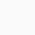# Precalculus: the Mathematics of Numbers, Functions and Equations

👤 Join WhatsApp Learners
Deal Score+1

Gain an introduction to the essential mathematical knowledge and skills required for your first calculus course.

### Join Email Learners

Follow the guide on landing page

## Get an introduction to precalculus fundamentals

This course is the first of two precalculus courses from the University of Padova that will provide you with an introduction to the fundamental mathematical skills required to complete your first course in calculus.

You will learn the basic precalculus required for college or undergraduate-level studies, including factoring and division, sets and set operations, reasoning and proofs, functions and graphs, and equations and inequalities.

In this online precalculus course, you will gain the foundation to support further mathematical studies and improve your use of maths in everyday life.

## What topics will you cover?

• Numbers: integers, rationals, real numbers, absolute value, induction.
• Functions: graphs, integer powers, roots and radicals, rational powers.
• Algebra: polynomials and their roots, quadratic polynomials, polynomial division, finding roots, binomial coefficients.
• Equations: types of equations, equivalence, polynomial equations, equations involving a radical, equations with several radicals/with absolute values, equations in several variables.
• Inequalities: linear and polynomial inequalities, inequalities involving radicals and absolute values.

## Who is the course for?

This course is for anyone who wants to brush up on their basic mathematical skills, particularly students in the last year of high school, or at the beginning of undergraduate studies, who intend to take the first course in calculus.

Precalculus: the Mathematics of Numbers, Functions and Equations

Originally posted 2021-12-24 12:45:26.

1